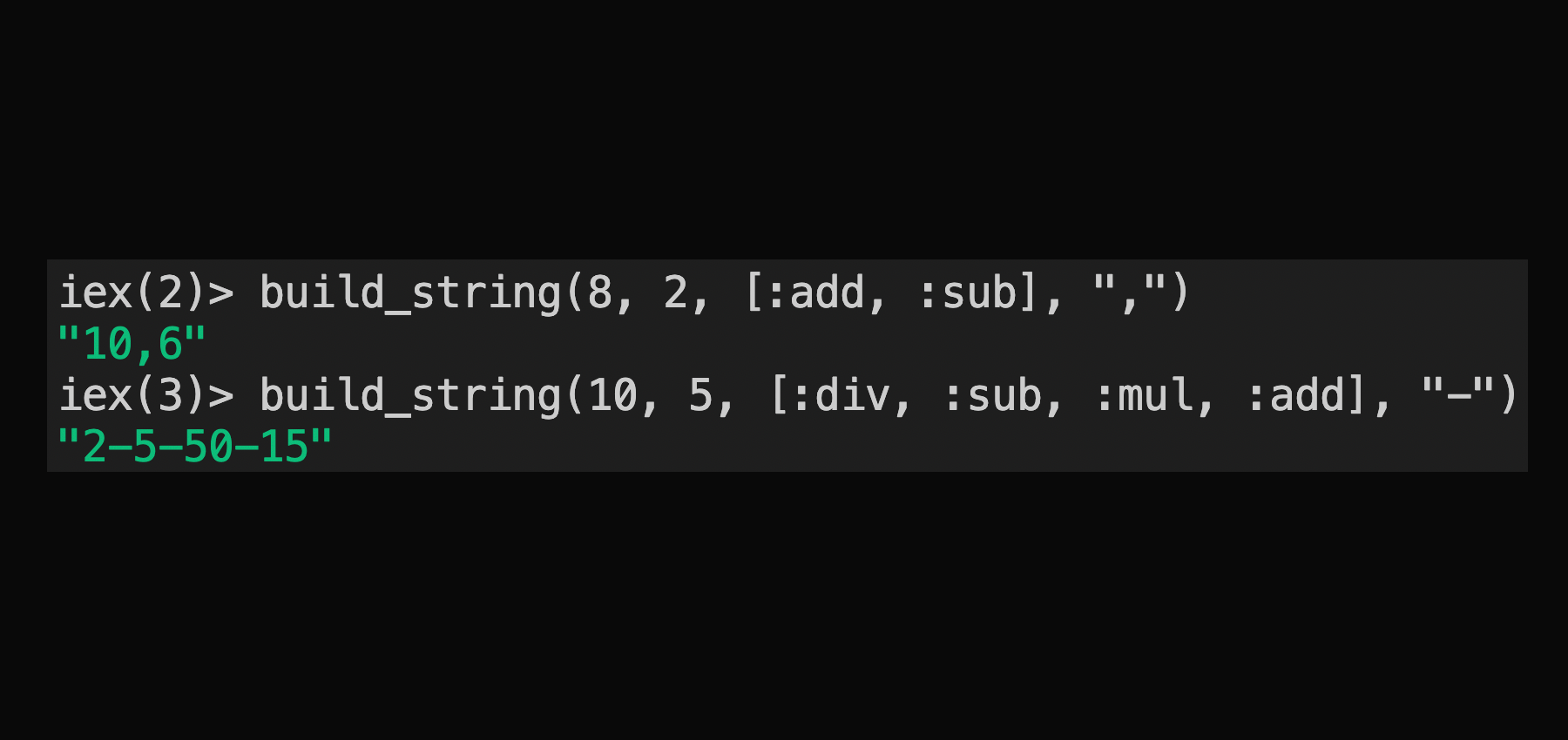Paraxial.io Blog

# Elixir code style, a brief example

by Michael LubasWrite a function in Elixir named `build_string` that takes 4 arguments:

``````a - An integer
b - An integer
ops - A list of the atoms [:add, :sub, :mul, :div] in any order, max length of 10
sep - A separator, such as "-" or ","``````

and returns a string where a and b are calculated, in-order, together according to the list of operations, delimited by the separator value. For example:

``````> build_string(8, 2, [:add, :sub], ",")
> "10,6"

> build_string(10, 5, [:div, :sub, :mul, :add], "-")
"2-5-50-15"``````

Here is an example of bad style:

``````defmodule Codex do

def build_string(a, b, ops, sep) do
Enum.reduce(ops, "", fn op, acc ->
acc <> sep <> Integer.to_string(calculate(a, b, op))
end)
end

def calculate(a, b, op) do
case op do
a + b
:sub ->
a - b
:mul ->
a * b
:div ->
div(a, b)
end
end
end``````

Focus on the `build_string/4` function:

``````  def build_string(a, b, ops, sep) do
Enum.reduce(ops, "", fn op, acc ->
acc <> sep <> Integer.to_string(calculate(a, b, op))
end)
end``````

From the start at `Enum.reduce`, the accumulator is a blank string, so reduce is creating a string. It is iterating over ops, so the accumulator will be built up in steps:

``````> build_string(8, 2, [:add, :sub], ",")
> # inside Enum.reduce
> "" <> "," <> "10"
> ",10"
> ",10" <> "," <> "6"
> ",10,6"``````

Then there is a call to trim the leading `sep`, in this case `","`. The call to `String.trim_leading/2` here is a red flag that there is a special case in the code evaluation, an additional leading separator value that should not have been introduced.

Now consider this version of `build_string/4`:

``````  # Good style
def build_string(a, b, ops, sep) do
ops
|> Enum.map(&calculate(a, b, &1))
|> Enum.join(sep)
end``````

`Enum.map` conveys that `calculate/3` transforms every element in the list, preserving the order. Elixir provides `Enum.join`, but even if it was not included, writing a function to join all the elements of the list together with a separator value makes this much more clear.

``````> build_string(8, 2, [:add, :sub], ",")
> [10, 6] # Produced by Enum.map([:add, :sub], &calculate(8, 2, &1))
> "10,6"  # Produced by Enum.join([10, 6], ",")``````

This avoids the problem of building up a string where the leading separator needs to be removed. The problem given may seem trivial, and it is. Making your code as easy to read as possible, by avoiding unnecessary cases, is the important point.

Paraxial.io is the only application security and compliance platform made for Elixir.

Subscribe for new posts about Elixir and Phoenix security.